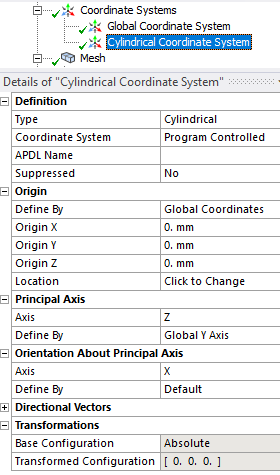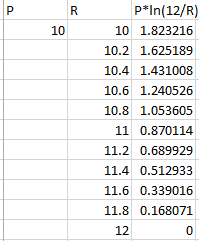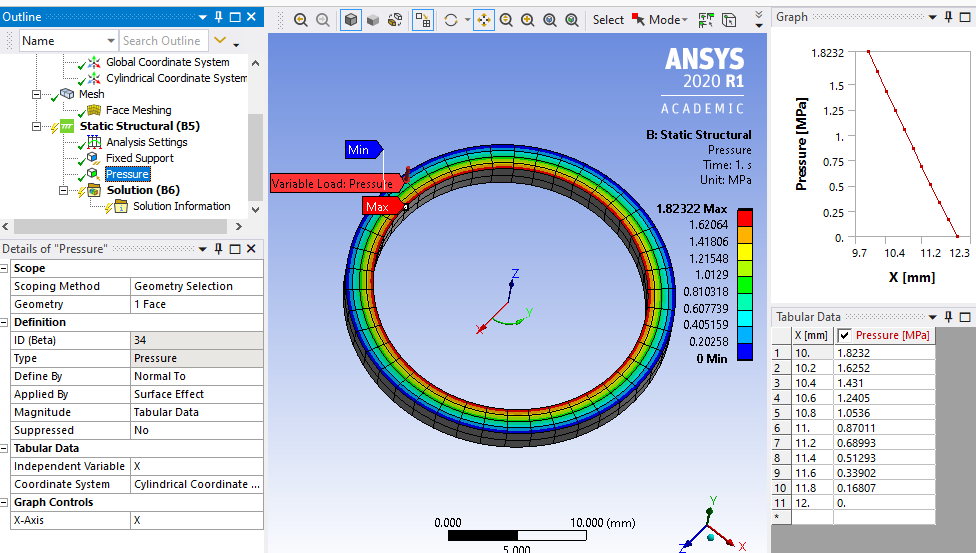peteroznewman
Subscriber
Thank you for a completely described question! You can get what you want without resorting to APDL.n#1. Make a Cylindrical Coordinate Systemn#2. Use Excel to make a table of Pressure vs Radius. In the example below, I put a P in front of your equation.n#3. Create a Pressure load using Tabular Data. Set the Independent Variable to X instead of Time. Use the Cylindrical Coordinate System and copy/paste the Excel cells into the Tabular Data field. Just pick the first cell and Paste.nStatic Structural is the right place to build this model. Transient Structural is useful if accelerations are high enough to generate significant inertia forces.n